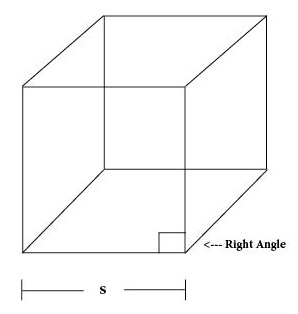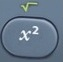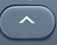# Cube

Cube = Three-Dimensional Shape with Six Square Faces (with all right angles)Basics
s = Side
SA = Surface Area = area covering all surfaces of cube (measured in square units)
V = Volume = space occupied by cube (measured in cubic units)
Right Angle = 90°

All of the angles in a cube are right angles.
A cube is a special version of a right rectangular prism in which all faces are equal.

The math involving the geometric cube often requires the calculation of arithmetic squares, square roots, cubes, and cube roots.is the squaring command.is the square root command.activatesabove.3   is the cubing command.

3is the cube root command.activatesabove.

Formulas (NOT on Mathematics Formula Sheet)

SA = 6s²
s = SA÷6

V = s³
s = ³V

Question
The side of an ice cube is 2.5 centimeters.  What is its surface area?

37.5 square centimeters

SA = 6s²
SA = 6 × 2.5² = 37.5

Input Display Commentblinker clears screen
6 × 2.56*2.5² SA = 6s²37.5 Answer
###### Cube

Question
The side of an ice cube is 2.5 centimeters.  What is its volume?

15.625 cubic centimeters

V = s³
V = 2.5³ = 15.625

Input Display Commentblinker clears screen
2.53 2.5³ V = s³15.625 Answer
###### Cube

Question
The surface area of a large ice cube is 216 square centimeters.  How long is its side?

6 centimeters

s = SA÷6
s = 216÷6 = 6

Input Display Commentblinker clears screen216 ÷ 6 216÷6

s = √SA÷66 Answer
###### Cube

Question
The volume of a large ice cube is 343 cubic centimeters.  How long is its side?

7 centimeters

s = ³V
s = ³343 = 7

Input Display Comment343 ³343 s = ³V7 Answer
###### Cube

Question
The surface area of a large ice block is 13824 square centimeters.  What is its volume?

110592 cubic centimeters

s = SA÷6
s = 13824÷6 = 48

V = s³
V = 48³ = 110592

Input Display Commentblinker clears screen13824 ÷ 6 13824÷6
s = √SA÷648 s
483 48³ V = s³110592 Answer
###### Cube

Question
The volume of a large ice cube is 729 cubic centimeters.  What is its surface area?

486 square centimeters

s = ³V
s = ³729 = 9

SA = 6s²
SA = 6 × 9² = 486

Input Display Comment729 ³729 s = ³V9 s
6 × 96*9² SA = 6s²486 Answer
###### Cube

Practice – Questions
1.  The side of an alphabet cube is 8.7 centimeters.  What is its surface area?

2.  The side of an alphabet cube is 8.7 centimeters.  What is its volume?

3.  The surface area of a large alphabet cube is 3750 square centimeters.  How long is its side?

4.  The volume of a large alphabet cube is 4096 cubic centimeters.  How long is its side?

5.  The volume of a large alphabet cube is 6859 cubic centimeters.  What is its surface area?